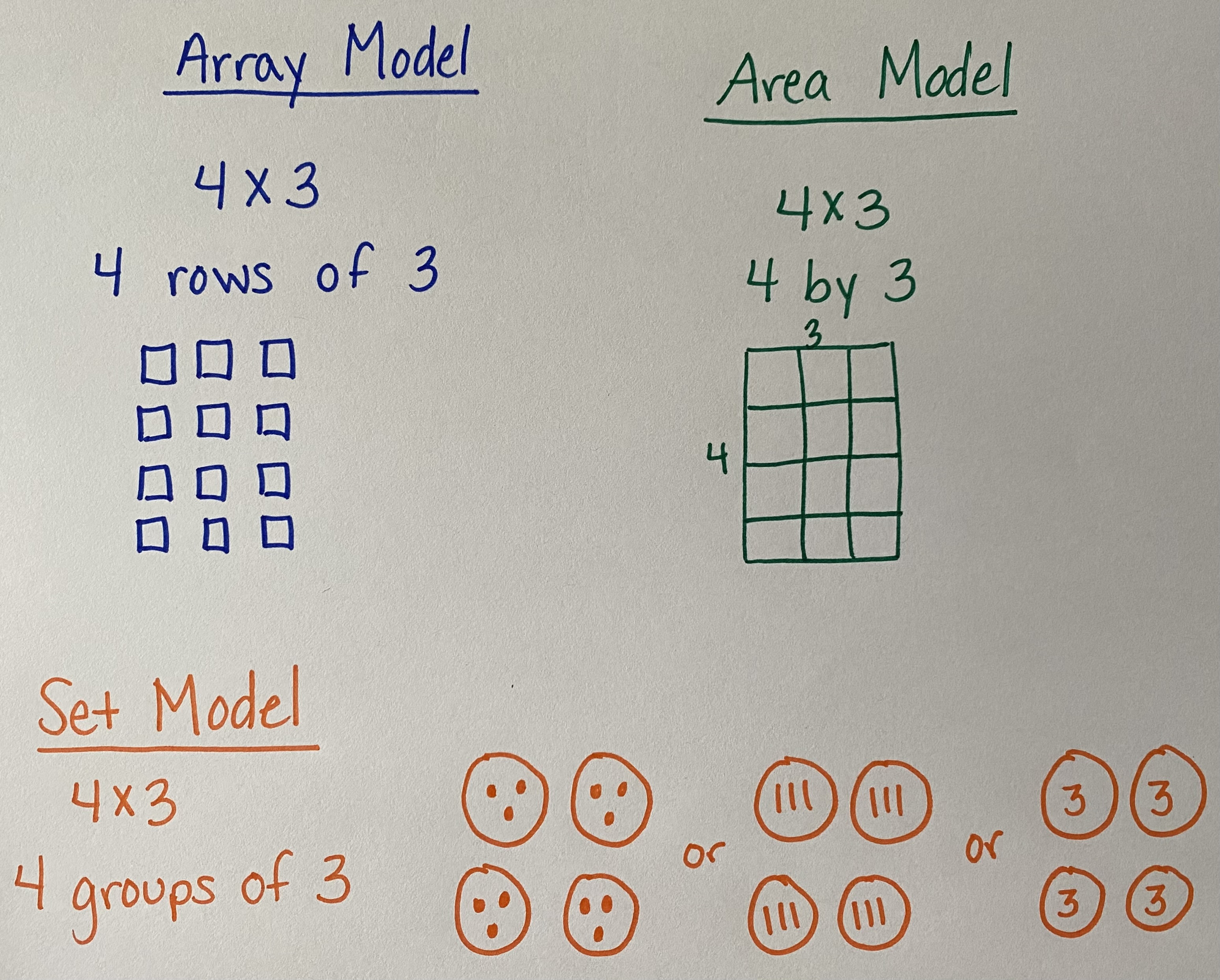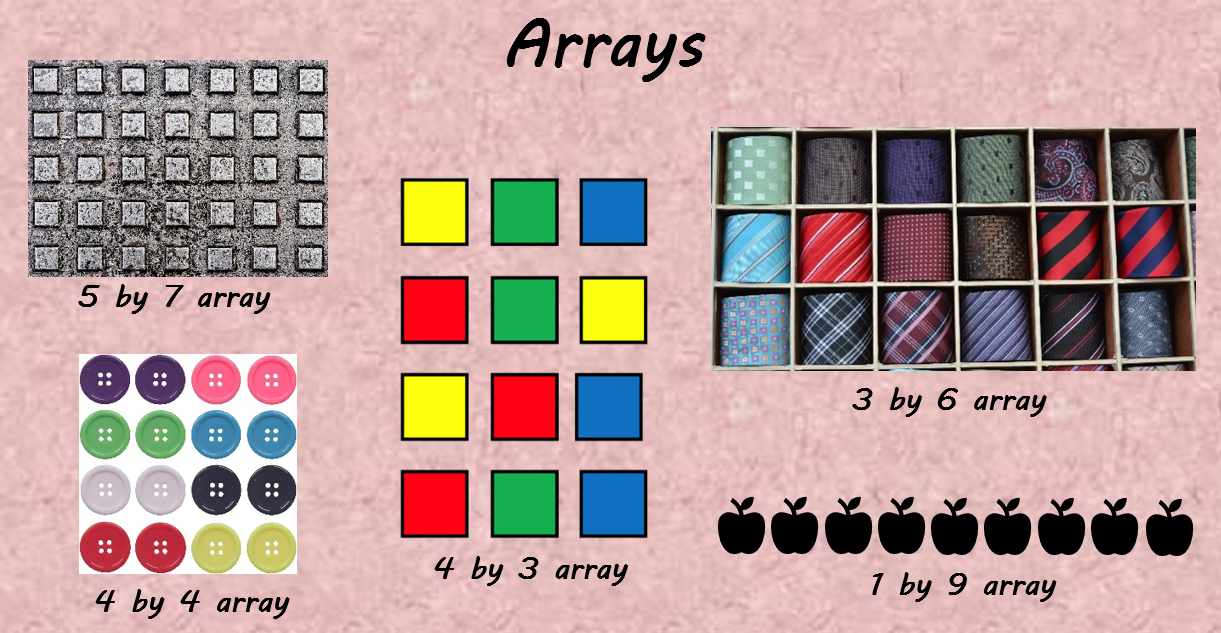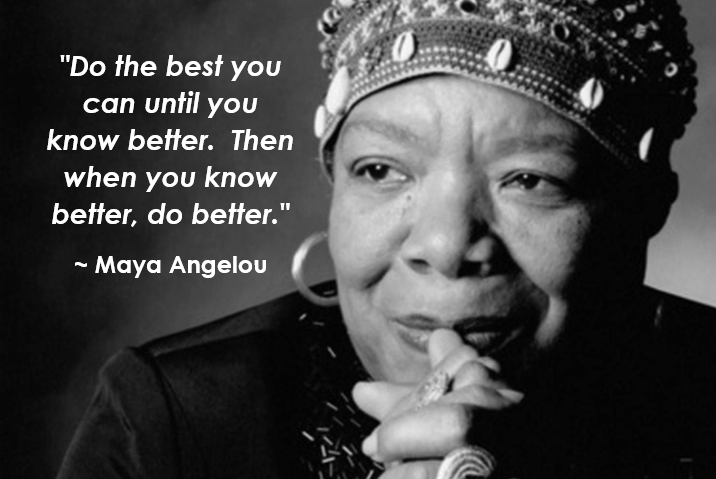# Multiplication Strategies Progression

#### Multiplication Strategies

Strategies are listed from the earliest strategies up through the standard algorithm.  Many are used side by side, but it is important to understand that the variety of strategies are used to build a deeper conceptual understanding and move to a more procedural model backed by conceptual understanding of multiplication.  Keep in mind that mastery of the standard algorithm of multiplication is not expected until grade 5 per the Maine Learning Results and Common Core State Standards, however students will begin practicing the standard algorithm along side other strategies much earlier than grade 5.

##### ModelsThere are many models for multiplication, all showing a variety of ways to show a number of equal groups.  When students first start working with multiplication they may use square tiles to build arrays or small quantities they can build out of manipulatives.  They then will transfer their models to drawings of equal groups potentially using tally marks, dots, and as they move to larger quantities, they may use digits to represent the groups - this transitions nicely to repeated addition or they may move to an area model.  The context of the problem will drive the type of model that will help students make sense of the problem.  As a reminder, students need varying types of problems (missing factor - number of groups, missing factor - missing quantity within a group, missing product - total) to practice their flexibility within multiplication.  These different types of problems should be introduced at different times within their learning progression.  For more information about varying the types of multiplication word problems students should use, check out the Glossary Table 2 from the Common Core State Standards for Mathematics.

One of the early strategies used in multiplication is repeated addition.  As students learn about equal groups they begin adding the same addend over and over (repeated addition).  7 boxes of 5 pencils may look like 5 + 5 + 5 + 5 + 5 + 5 + 5 = 35 pencils.  Students may begin to notice that the repeated addition can seem much like skip counting by the number being added.  7 boxes of 5 pencils may sound like 5, 10, 15, 20, 25, 30, 35 pencils.  Students would need to keep track of each 5 they are skip counting by until they get to the 7 times or 7 boxes of pencils.  As students look for more efficient strategies, they begin to know from memory some of their facts and use these in other strategies.

##### Arrays (Models)An array model form multiplication is a physical or visual model demonstrating equal row.  Students can use physical objects or manipulatives or they may draw shapes to model the multiplication problem they are solving.  This strategy builds nicely into helper facts, doubling, and the area model.

##### Helper Facts and Doubling

Using helper facts allows students to build on their known facts making more challenging problems easier to solve.  Students typically learn their 2s, 5s, and 10s facts first.  They have been counting by 2s, 5s, and 10s since kindergarten.  Using those facts, they can build to more complex problems.  To solve 6 x 7, a student may think of the problem like 6 groups of 7 and if they know that 5 groups of 7 or 5 x 7 = 35, then they know they need one additional group of 7 to 35 + 7 = 42 so 6 x 7 = 42.

Doubling is a similar strategy using a helper fact or known fact that is half of the original problem.  For example, 4 x 9 may be challenging to think about, but since students are more familiar with 2s, 5s, and 10s facts, they might know 2 x 9 = 18.  Thinking that 2 x 9 = 18 is half of 4 x 9, they can then double 18.  Students may show this as 18 x 2 or 18 + 18.  What is actually happening mathematically is 4 x 9 is broken into (2 x 9) + (2 x 9), which is equal to 36.  Please see the video for another example that uses doubling and the area model to explain.

##### Area Model (Connects to Division)

The area model for multiplication uses a visual rectangular representation to show the area and can be broken up in many ways to help solve larger and smaller multiplication problems.  Students can use base ten blocks to physically build the area model, then transitioning into pictorial representations with some base ten detailing, then into less detailed representations.  This leads nicely into the area model for division.  To see the area model demonstrated, check out the video.

##### Partial Products

The area model leads nicely into the partial product strategy.  Much like addition, partial products breaks factors, or numbers being multiplied, into their place values or expanded form.  To start partial products, students can use an area model to visualize where each partial product comes from.  As students build their understanding of partial products, they will be able to use this strategy without the area model, though they may need to continue to go back to the area model as the numbers they are working with expand to more than two digits.

##### Lattice Multiplication

Lattice multiplication is a strategy that, when taught with the proper place value supports, students can understand why this strategy works.  When taught after an understanding of partial products, students may be able to make connections amongst the strategies.  It can seem like a set of steps, or procedural process, much like the standard algorithm, that is why place value conversations are important.

##### US Standard Algorithm

The standard algorithm is the strategy most people think of when asked to multiply larger numbers.  They is the strategy we all learned as we learned about multiplication.  As a reminder, mastery of the standard algorithm for multiplication is a grade 5 standard expectation, however students should be introduced to it before grade 5.  Once students have conceptually learned what multiplication is, can use a variety of strategies, the procedural steps of the standard algorithm should have a connection.  Students can even compare the strategy of partial products to the standard algorithm and compare where they see connections in the strategy.

#### Graham Fletcher Video - Progression of Multiplication